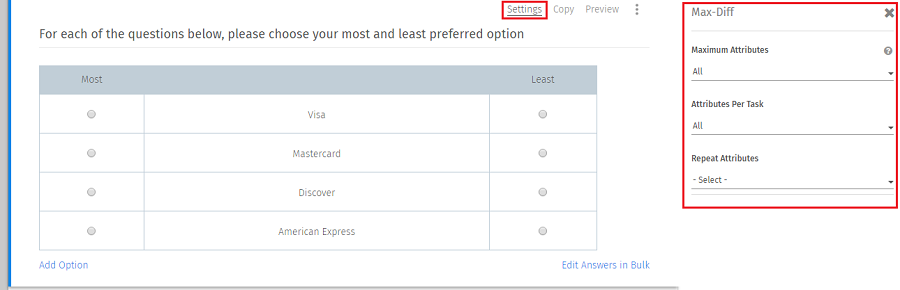# Max Diff Scaling - Frequently Asked Questions

## Can I set up an experimental design - where the total items are larger than the items presented in a single screen?

Yes. You can change the 'Maximum Attributes' and 'Attribute Per Task' to control the number of items displayed in a single screen.

Maximum Attributes: If you have numerous attributes then you can limit the attributes displayed on the screen. For example if you have 9 attributes and you set “6” in the drop down this mean the respondent will only see 6 attributes out of list of 9. If you set drop down values as “All” this mean all the attributes (i.e. 9) will be tested.

Attribute per task: Out of selected maximum attribute we can select how many attributes we want to show per task. Example: if you set Attributes per task as “3” and Maximum Attributes as “All” then you will have 3 cycles.Logit.

## How is the Preference Share calculated

1. Raw Utility (Best)

U(b) = Ln(B(i) / 1 - B(i)))

2. Raw Utility (Worst)

U(w) = -Ln(W(i) / 1 - W(i))

3. Raw Utility

Raw Utility [U(i)] = Average(U(b) , U(w))

4. Scaled

U(Scaled)(i) = U(i) - Average(U)

5. Transformed

U(Transformed)(i) = EXP(U(Scaled)(i))

6. Preference Share

Preference Share = U(Transformed)(i) / SUM(U(Transformed))

Note: Back Button is not supported in Max-Diff Question.